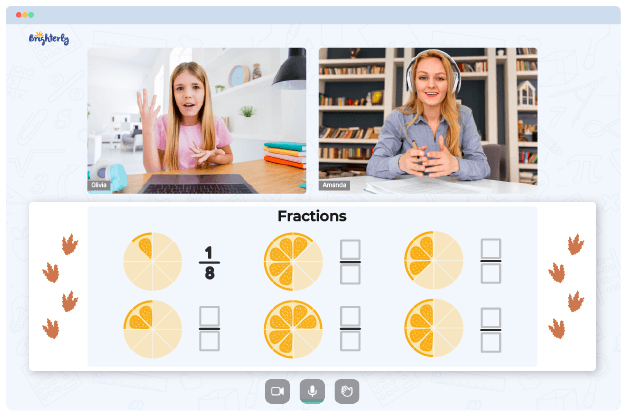# Percentage Worksheets

A percentage is an essential aspect of mathematics. The knowledge of this concept helps students grasp the monetary realities of everyday life — discounts at stores, inflation rates, bank interest rates, and statistical averages.

## Free Percentage Worksheet

Generally, numbers expressed as percentages are more straightforward compared to those expressed as fractions. In mathematics, a percentage is a ratio or number expressed as a fraction of 100. Two components comprise a fraction: the numerator and the denominator, representing an entire part.

A percentage is dimensionless; it has no unit of measurement. A percent worksheet provides students with experience in calculating the percent value by multiplying the numerical value of the ratio by 100. In the past, calculations were done using multiples of 1/100.

Top professionals design Brighterly’s percentages worksheets to ensure that the tools make learning seamless for young scholars. With these worksheets, it is only a matter of time before your kids become experts in calculating percentages.

## Benefits of Percent Worksheets

There are numerous benefits of studying with percentage worksheets. Below are some of them.

Math for Kids

Is Your Child Struggling With Math?
1:1 Online Math Tutoring### Support of independent study

Students will find pleasure in studying independently with a worksheet. Usually, worksheets contain answers to the questions; hence, kids will know when they are wrong. These answers will further quicken learning, relearning, and mastery of the topic.

### Effective time management

Students can improve their time management skills. The ability to quickly and accurately solve mathematical problems is crucial. Also, students will promptly ascertain areas they need more understanding of by working through practice problems in the worksheets.

### Everyday application

A wide variety of situations call for an understanding of percentages. By studying with a worksheet, students will have sufficient knowledge of percentages and the ways to apply them in everyday life.### Percentage Worksheets PDF

Percentages Worksheets### Percentage Worksheets PDF

Percent Worksheets### Percentage Worksheets PDF

Percentages Worksheet### Percentage Worksheets PDF

Percent Worksheet

## Printable Percentage Worksheets

You can download printable percentage worksheets from the Brighterly’s website to allow your kids to study independently. The printable version of these worksheets is more accessible, hence easier to use.

### More Percents Worksheets

Trouble with Fractions?• Does your child need extra support with understanding fractions?
• Start lessons with an online tutor.

Is your child finding it hard to master the concept of fractions? An online tutor could be of help.